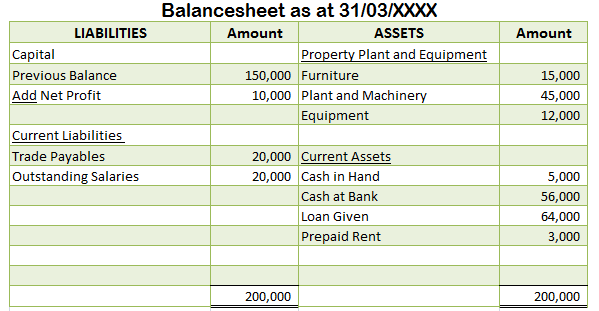# What is the formula for working capital?

-This question was submitted by a user and answered by a volunteer of our choice.

## Working capital = Current Assets – Current Liabilities

I believe if you want to understand how the above formula works you will need to understand each part of this formula i.e you will first need to have a clear understanding of the concept of current assets and current liabilities.

Current assets

It refers to all the assets including cash and cash equivalents which are expected to be converted within a year or within the operating cycle of an entity if such entity has an operating cycle longer than a year.

Current liabilities

It refers to all the payables or debts which an entity expects to discharge within a year or the operating cycle of such entity provided such an entity has an operating cycle longer than a year.

I understand that even though you got a rough idea of this concept you may not be confident while applying this concept hence, to relate to this concept a numerical example would be of great help.

So, I believe once you have a look at the below-mentioned example you will be in a better position to comfortably apply this formula:Here, Working Capital = Current Assets – Current Liabilities

= Cash in Hand + Cash at Bank + Trade Receivables + Prepaid Rent –Trade Payables  –                  Outstanding Salaries

= 5,000 + 56,000 + 64,000 + 3,000 – 20,000 – 20,000

= 128,000 – 40,000

= 88,000.

It will add more value to your understanding if you also interpret the concept of Gross Working Capital and Net Working Capital.

Gross Working Capital

Gross working capital is the sum total of current assets of an entity. It includes

• Cash and Cash Equivalents
• Inventory
• Short term Investments
• Other Marketable Securities.

Net Working Capital

Now, the net working capital of an entity is nothing but the working capital of an entity. In simple terms, it is a difference between the current assets and current liabilities of an entity.

>Related Long Quiz for Practice Quiz 33 – Working Capital

###### Subscribe
* indicates required